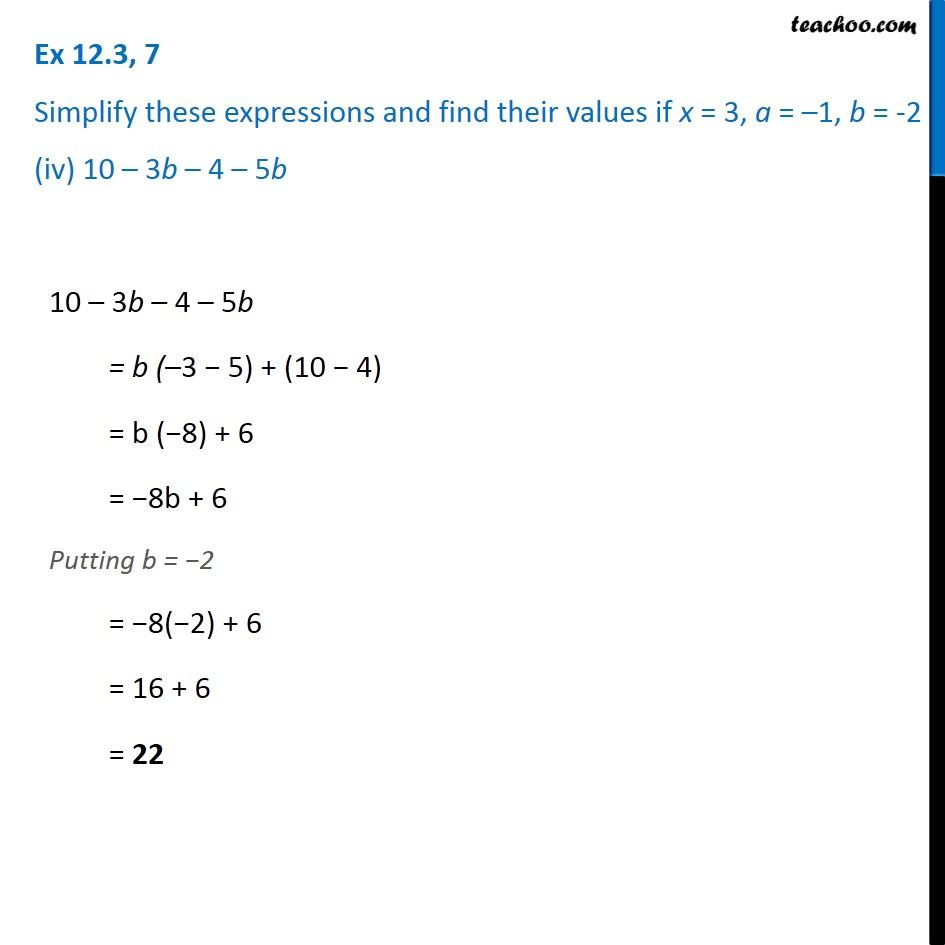Ex 12.3

Chapter 12 Class 7 Algebraic Expressions
Serial order wiseGet live Maths 1-on-1 Classs - Class 6 to 12

### Transcript

Ex 12.3, 7 Simplify these expressions and find their values if x = 3, a = –1, b = -2 (iv) 10 – 3b – 4 – 5b 10 – 3b – 4 – 5b = b (–3 − 5) + (10 − 4) = b (−8) + 6 = −8b + 6 Putting b = −2 = −8(−2) + 6 = 16 + 6 = 22